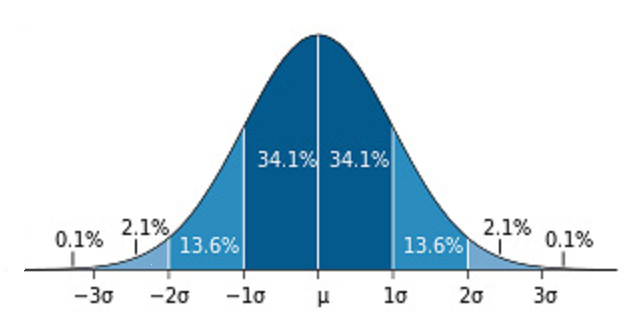Today May 12, 2023.......... 1.1K unique readers ...... 12K requests.......... official Cloudflare stats ..........

# The standard normal curve - how to use

### The standard normal curve - how to use#### What is the standard normal curve?

The standard normal curve is a curve that can be generated by a function, an equation. It is bell-shaped, symmetrical, its mean is 0, and it has three standard deviations. The equation that creates the standard normal curve is:
$$y={{1\overσ\sqrt{2π}}}e^{-{(χ-μ)^2}\over2σ^2}$$

#### How is the standard normal curve used

Normal distribution, use number 1. To describe, to organize data
Normal distribution, use number 2. Making statements of probability, betting
Normal distribution, use number 3. To make statements regarding the .........Next
Rate it: